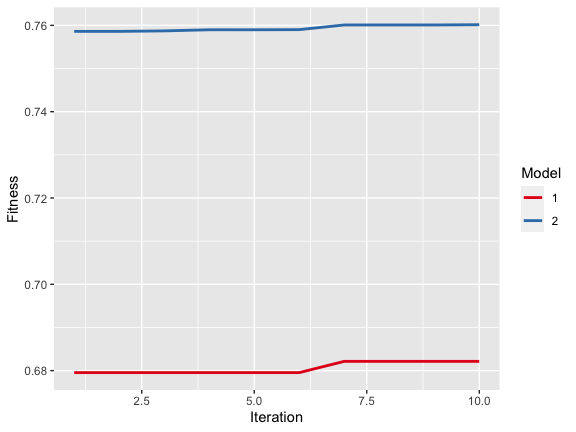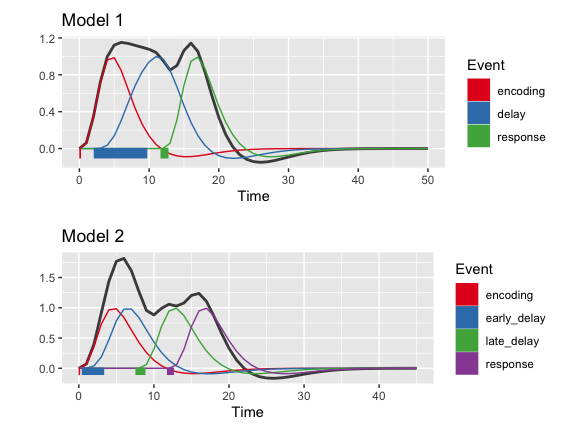# An example on how to use automated model parameter search

#### 2023-02-15

In this example we show how you can use the autohrf package to automatically generate data-informed event models for general linear modeling of task-based fMRI data. Let us start this example by loading required libraries and the data from the spatial working memory study.

# libraries
library(autohrf)

df <- swm
head(df)
##   roi t          y
## 1 L_1 0 0.02712162
## 2 L_1 1 0.06248649
## 3 L_1 2 0.12908108
## 4 L_1 3 0.30183784
## 5 L_1 4 0.51691892
## 6 L_1 5 0.65970270

The loaded data frame has 11520 observations, each with 3 variables (roi, t, and y) roi denotes the region of interest, t the time stamp and y the value of the BOLD signal. Note that input data for the autohrf package should be always organized in this manner.

Next, we define two different model constraints, which will be used by the autohrf function to find the best fitting model given these constraints.

# model constraints for three event predictors
model1 <- data.frame(event = c("encoding", "delay", "response"),
start_time = c(0, 0.15, 10),
end_time = c(0.15, 10, 13))

# model constraints for four event predictors
model2 <- data.frame(event = c("encoding", "early_delay", "late_delay", "response"),
start_time = c(0, 0.15, 5, 10),
end_time = c(0.15, 5, 10, 13))

# join different model constraints
models <- list(model1, model2)

Once we define the constraints for our models we can use the autohrf function to automatically find model parameters that fit our data best. Note that this is only an illustrative example and the set amount of the population size and iterations of the genetic algorithm is way too small for any kind of proper analysis.

# to speed vignette building we here load results from a previous autohrf run
autofit <- swm_autofit

# in practice you should run
# autofit <- autohrf(df, models, tr = 1, population = 10, iter = 10)

When the automated fitting process is completed, we can use the plot_fitness function to check whether our model solutions converged.

# plot models' fitness across iterations
plot_fitness(autofit)Next, we can use the get_best_models function to extract the best model for each of the provided constraints.

# return automatically derived parameters
best <- get_best_models(autofit)
##
## ----------------------------------------
##
## Model 1
##
## Fitness:  0.682151
##
##      event start_time duration
## 1 encoding       0.09     0.04
## 2    delay       2.16     7.48
## 3 response      11.73     0.95
##
## ----------------------------------------
##
## ----------------------------------------
##
## Model 2
##
## Fitness:  0.7601532
##
##         event start_time duration
## 1    encoding       0.06     0.02
## 2 early_delay       0.48     2.80
## 3  late_delay       7.63     1.15
## 4    response      11.83     0.74
##
## ----------------------------------------

Based on calculated fitness scores we can see that the second model fits our data better. Furthermore, we can use the plot_best_models function to visually inspect the best models.

# visualize automatically derived parameters
plot_best_models(autofit)